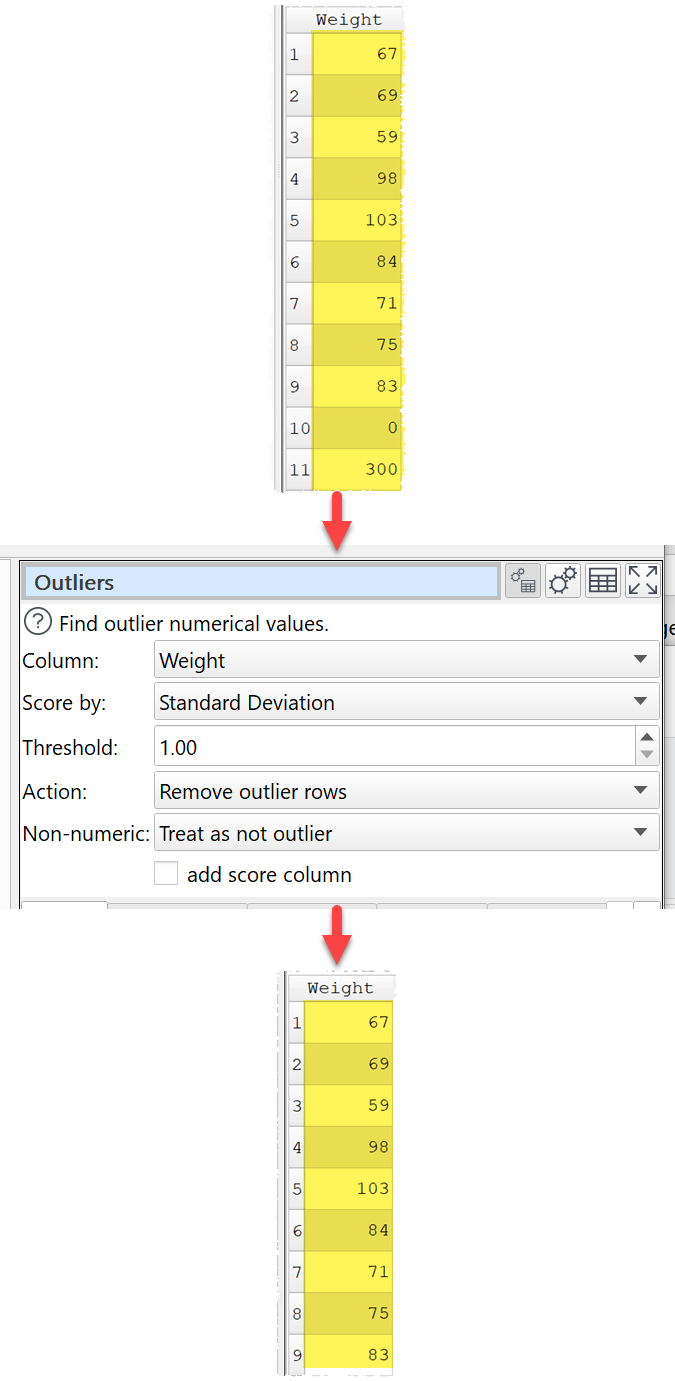﻿ Reference > Transforms > Outliers

# Outliers

## Description

Find outlier numerical values.

## Examples

Set outliers empty based on quartiles and add a column with the outlier score:Remove rows with values that are more than 1 Standard Deviation from the mean:One.

## Options

Select the column you wish to check for outliers.

Set Score by depending on how you wish to score outliers.

oInter Quartile Range scores values on how far they are above Q3 or below Q1 as proportion of the Inter Quartile Range. For example:

A value 2 IQRs above Q3 scores 2.0.

A value 1 IQR below Q1 scores -1.0.

A value between Q3 and Q1 scores 0.0.

oStandard Deviation scores values on how many deviations they are from the average (mean). This is also known as a Z-score. For example:

A value 2 Standard deviations above the average scores 2.0.

A value 1 Standard deviations below the average scores -1.0.

Any score that is greater than Threshold or less than -Threshold is considered an outlier. E.g. if the threshold is 1.5 then values that scores more than 1.5 or less than -1.5 are considered outliers. The corresponding upper and lower 'fence' values are shown in the Info tab.

Set Action to the action to take for outliers:

oChange to threshold: Value greater than the upper fence value or less than the lower fence value are set to the nearest fence value. Non-numeric values are not changed, as it isn't clear which fence value to set them to.

oRemove outlier rows: Rows with outlier values are removed.

oKeep outlier rows: Only rows with outlier values are kept.

oSet value: Outlier values are changed to the value provided in the Value field.

Set Non-numeric depending on whether you want to treat non-numeric values as outliers.

Check add score column if you want to add an extra column with the outlier scoring.

## Notes

Warnings are shown in the Warnings tab for non-numeric values.

Use Num Format to change the format of numbers (e.g. remove group separators).

Use Impute to impute any missing values.

Use Sort to sort by values in the score column.

You can convert dates into numerical values (e.g milliseconds since 1970 or Julian day) using Calculate or Javascript.